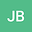The Propagation of Hermite-Gauss wavepackets in Optics and Quantum Mechanics
•## Abstract

The two-dimensional paraxial equation of optics and the twodimensional time-dependent Schr odinger equation, derived as approximations of the three-dimensional Helmholtz equation and the three-dimensional time-independent Schr odinger equation respectively, are identical. Here the free propagation in space and time of Hermite-Gauss wavepackets (optics) or Harmonic Oscillator eigenfunctions (quantum mechanics) is examined in detail. The Gouy phase is shown to be a dynamic phase, appearing as the integral of the adiabatic eigenfrequency or eigenenergy. The wave packets propagate adiabatically in that at each space or time point they are solutions of the instantaneous harmonic problem. In both cases, it is shown that the form of the wave function is unchanged along the loci of the normals to wave fronts. This invariance along such trajectories is connected to the propagation of the invariant amplitude of the corresponding free wave number (optics) or momentum (quantum mechanics) wavepackets. It is shown that the van Vleck classical density of trajectories function appears in the wave function amplitude over the complete trajectory. A transformation to the co-moving frame along a trajectory gives a constant wave function multiplied by a simple energy or frequency phase factor. The Gouy phase becomes the proper time in this frame.
29 May 2023Submitted to Natural Sciences
30 May 2023Submission Checks Completed
30 May 2023Assigned to Editor
31 May 2023Reviewer(s) Assigned
20 Jul 2023Review(s) Completed, Editorial Evaluation Pending
20 Jul 2023Editorial Decision: Revise Minor